# Input

• The first line contains an integer T, the number of test cases. Then the test cases follow.
• Each test case contains a single line of input, four integers D,d,P,Q.

• 1≤T≤10⁵
• 1≤d≤D≤10⁶
• 1≤P,Q≤10⁶

# Sample Input

`32 1 1 13 2 1 15 2 1 2`

`3413`

# Explanation

• On the first day, the rate of production is 1 dollar per day so 1 dollar is printed on the first day.
• On the second day, the rate of production is 1+1=2 dollars per day so 2 dollars are printed on the second day.
• The total amount of money printed in 2 days is 1+2=3 dollars.
• For the first two days, the rate of production is 1 dollar per day so 1⋅2=2 dollars are printed on the first two days.
• On the third day, the rate of production is 1+1=2 dollars per day so 2 dollars are printed on the third day.
• The total amount of money printed in 3 days is 2+2=4 dollars.
• For the first two days, the rate of production is 1 dollar per day so 1⋅2=2 dollars are printed on the first two days.
• On the next two days, the rate of production is 1+2=3 dollars per day so 3⋅2=6 dollars are printed on the next two days.
• On the last day, the rate of production is 3+2=5 dollars per day so 5 dollars are printed on the last day.
• The total amount of money printed in 5 days is 2+6+5=13 dollars.
`import java.util.*;import java.lang.*;import java.io.*;/* Name of the class has to be "Main" only if the class is public. */class Codechef{    public static void main (String[] args) throws java.lang.Exception    {        //SOLUTION        FastReader sc = new FastReader();        long t = sc.nextLong();        while(t-- > 0){        long D,d,p,q;        D = sc.nextLong();        d = sc.nextLong();        p = sc.nextLong();        q = sc.nextLong();        long n = D/d;        long sol=0;        sol= n*p*d + q*d*(n*(n-1))/2 + (D%d)*(p+n*q);        System.out.println(sol);        }    }    // Below are just the classes needed for fast Input in Java with Fast Reader    static class FastReader {        BufferedReader br;        StringTokenizer st;        public FastReader() {            br = new BufferedReader(new InputStreamReader(System.in));        }        String next() {            while (st == null || !st.hasMoreElements()) {                try {                    st = new StringTokenizer(br.readLine());                } catch (IOException e) {                    e.printStackTrace();                }            }            return st.nextToken();        }        int nextInt() {            return Integer.parseInt(next());        }        long nextLong() {            return Long.parseLong(next());        }        double nextDouble() {            return Double.parseDouble(next());        }        String nextLine() {            String str = "";            try {                str = br.readLine();            } catch (IOException e) {                e.printStackTrace();            }            return str;        }    }}`

--

--

--

## More from Anubhav Mishra

Software Developer ,currently pursuing B.Tech in Information Technology. Electronic Dance Music is love.Also like all phone related technologies

Love podcasts or audiobooks? Learn on the go with our new app.

## I will convert xd to html css bootstrap5 responsive figma, zeplin, pdf to html website## Restoring Archived Data with Splunk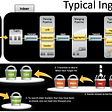## Bonny: Extending Kubernetes with Elixir — Part 3## 5 reasons why your project manager sucks## INTRODUCING THE METADEX ACCESS PASS (MAP)## Continuous Integration (CI) get easy more than ever with Github + TravisCI + GKE/GCR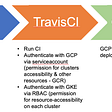## Java variables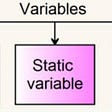## How to choose tech for your startup or app## Anubhav Mishra

Software Developer ,currently pursuing B.Tech in Information Technology. Electronic Dance Music is love.Also like all phone related technologies

## Randomized algorithms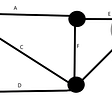## Main Classes and Interfaces for Multi-Threading in Java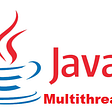## Polymorphism in JAVA